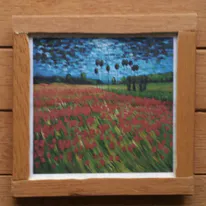# Making card games on micro:bit, Part 2

Learn how to use micro:bit to show playing cards on an SSD1306 128x64 OLED display. A key element of any card game.

IntermediateFull instructions provided1 hour997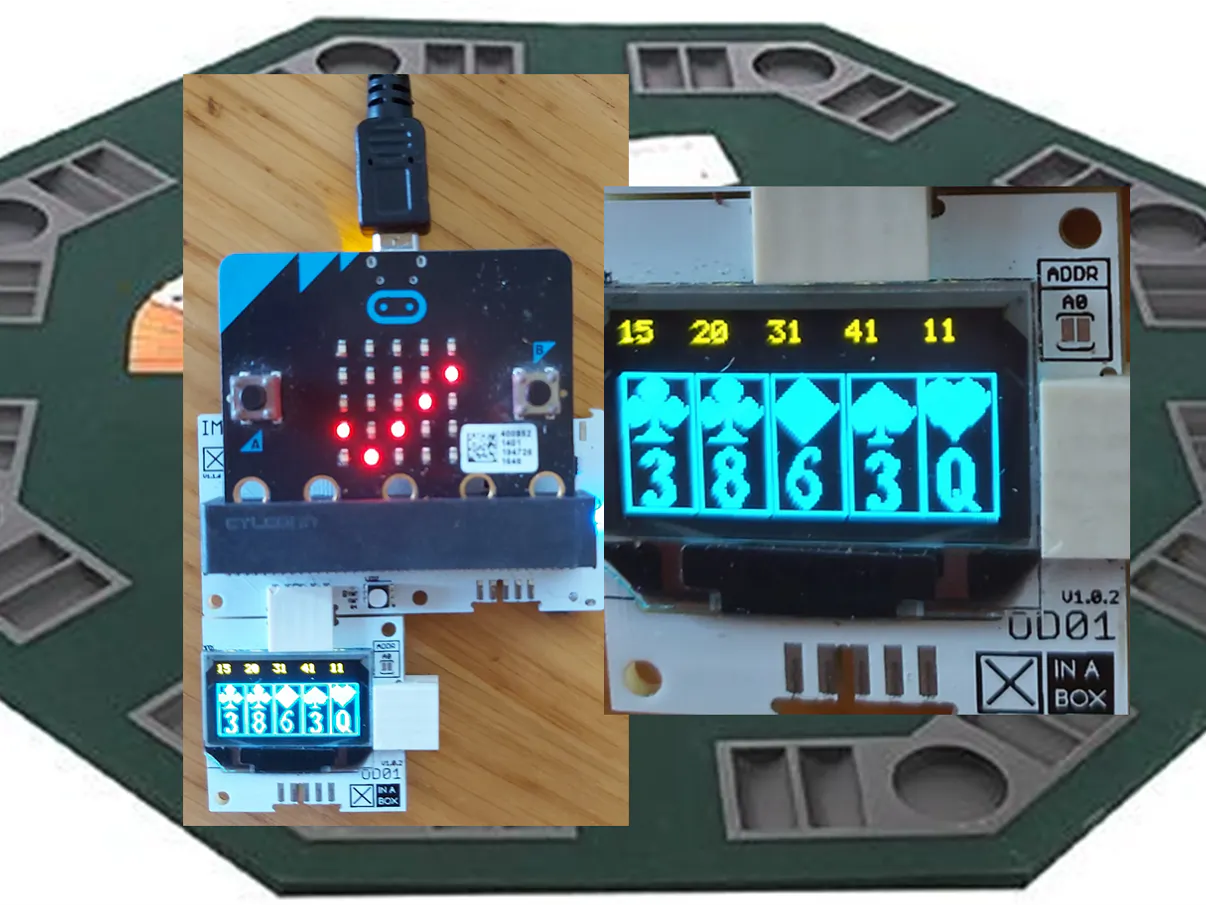## Things used in this project

### Hardware components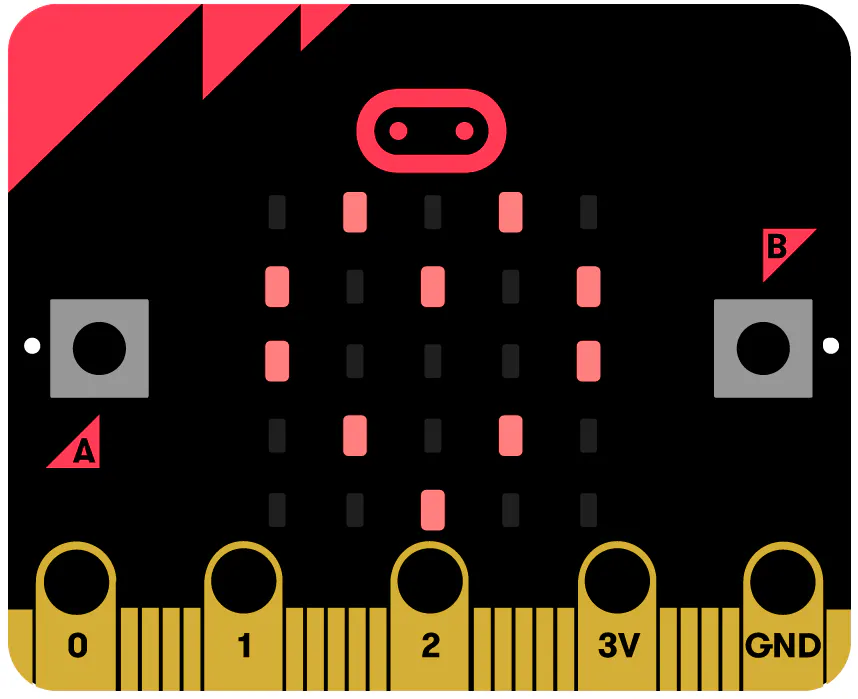BBC micro:bit board Any SBC that you can code in MakeCode will do - check out the excellent Maker.MakeCode IDE and see the broad range of boards supported :)
×1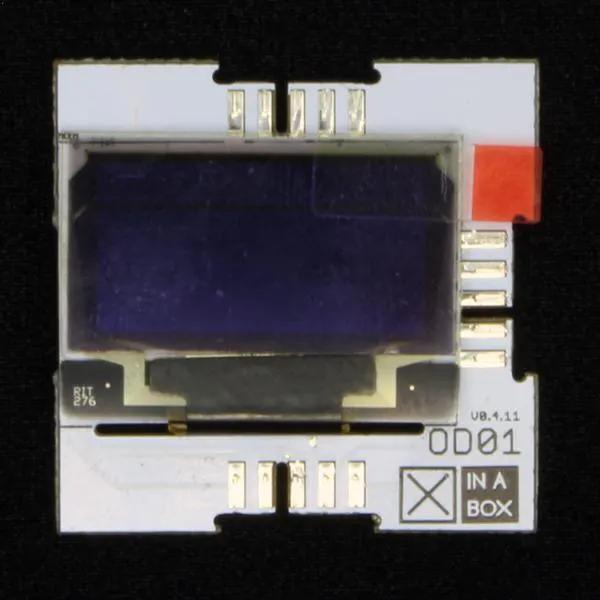XinaBox OD01 We use an SSD1306 as the display. You can use a different type of screen or a differently mounted SSD1306 - the code should be easy to adapt
×1
 XinaBox IM01 micro:bit bridge with microSD card support We use persistent memory to ensure our graphics are visually appealing. 17 small (1kb) bitmaps are saved on microSD card and referenced in runtime. This works around limitations in micro:bit memory,
×1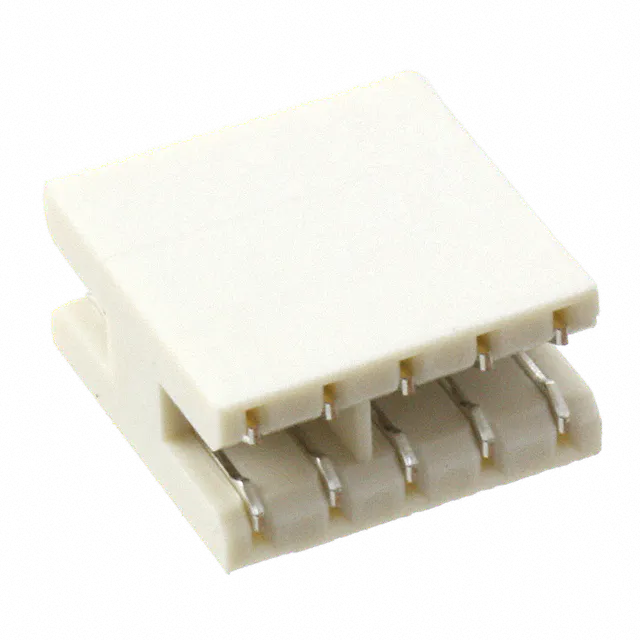XinaBox XC10 You only need 1 of these, to connect the OD01 to the IM01.
×1

## Schematics

### Guide to 'public' functions

Review the functions that we developed in this project and how they fit together. There are only 2 functions that you need to use, as explained on this diagram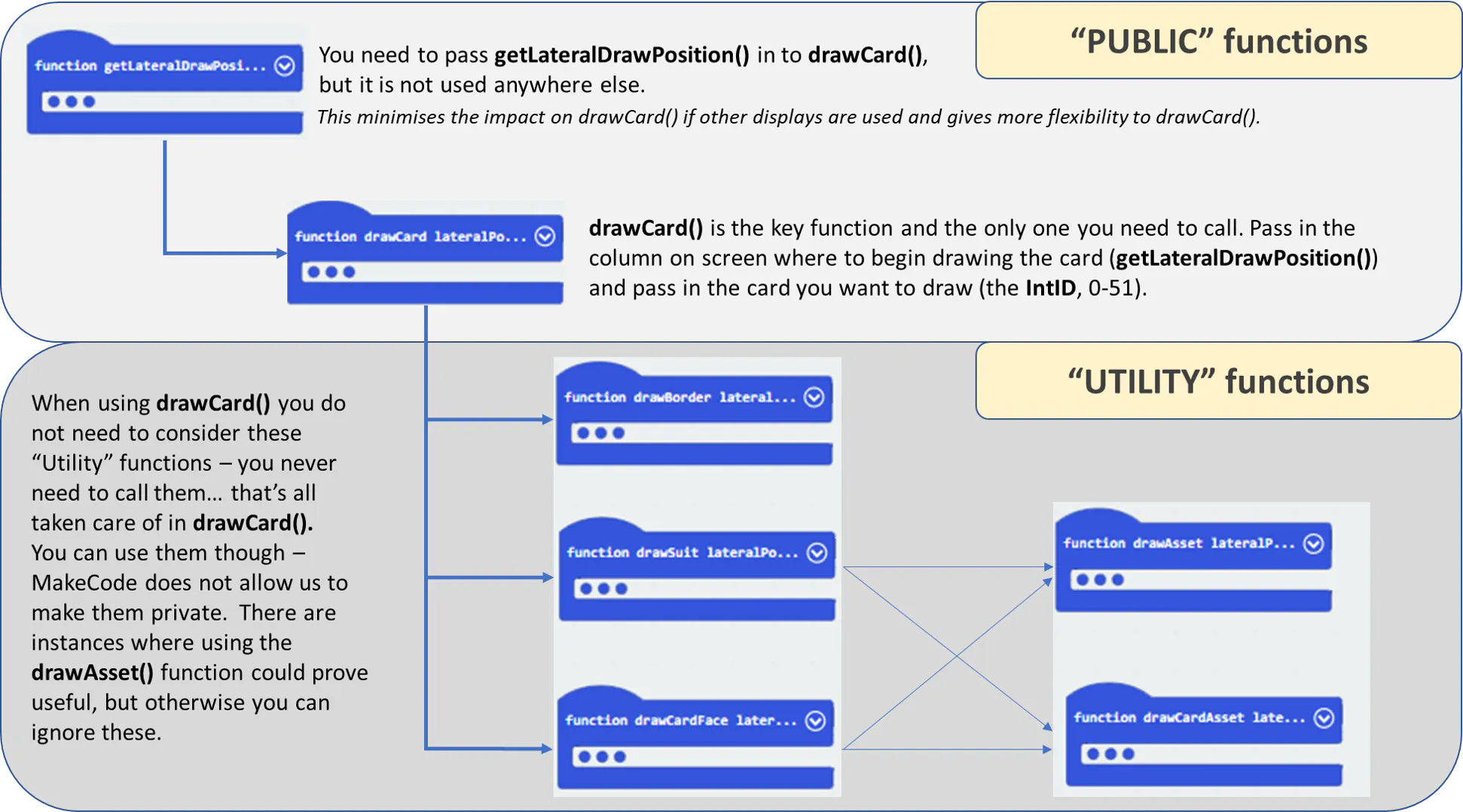### Lookup table

Each card in a standard deck is represented by an integer from 0 to 51 (called IntID). But convention: 0, 13, 26, 39 = Ace. +1 = two, +2 = three etc. 0-12 = hearts, 13-25 = Clubs etc. A lot of info is encoded into IntID - a lot of that info is shown in this lookup table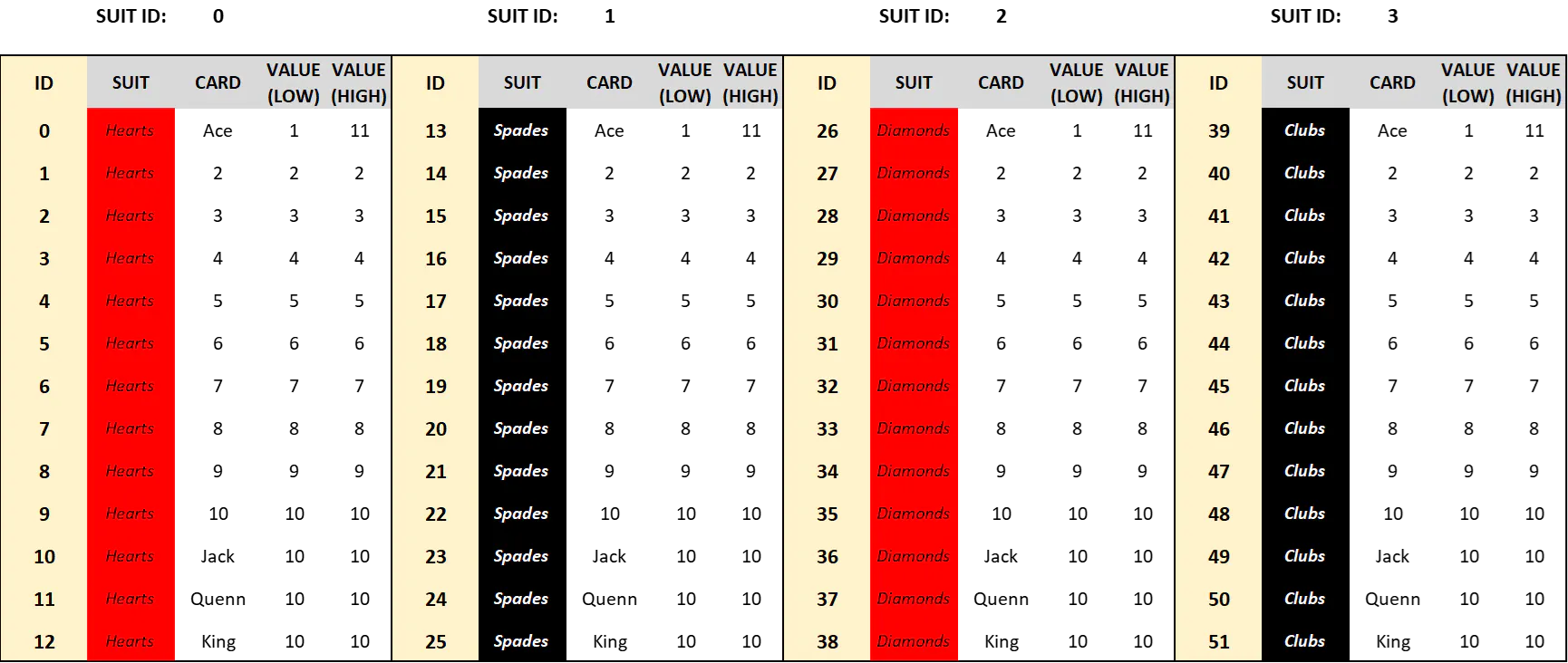## Code

### Displaying playing cards on an SSD1306

JavaScript
Ignore the warnings below - load the extensions (OD01 and IM01) and it will be fine. You are better off using the shared project link: https://makecode.microbit.org/_LXx2PWCa2P1m
```function drawCardFace (lateralPosition: number, faceID: number) {
drawCardAsset(lateralPosition + 1, 40, IM01.readFile("face" + convertToText(faceID) + ".txt"))
}
function drawAsset (lateralPosition: number, height: number, bitmapString: string, imageWidth: number, imageHeight: number) {
countColumns = 0
countRows = 0
for (let index = 0; index <= imageWidth * imageHeight - 1; index++) {
if (bitmapString.charAt(index + 1) == "1") {
OD01.pixel(lateralPosition + countColumns, height + countRows, 1)
}
countColumns += 1
if (countColumns >= imageWidth) {
countColumns = 0
countRows += 1
}
}
}
input.onButtonPressed(Button.A, function () {
OD01.clear()
for (let index2 = 0; index2 <= 4; index2++) {
basic.showNumber(index2)
drawCard(getLateralDrawPosition(index2), playerHand[index2])
OD01.showNumber(playerHand[index2], index2 * 26, 0, 1)
}
basic.showIcon(IconNames.Yes)
})
function shufflePack () {
resetPack()
for (let loopX = 0; loopX <= packSize - 1; loopX++) {
cardChosen = randint(0, originPack.length - 1)
deckOfCards.insertAt(loopX, originPack.removeAt(cardChosen))
}
activeCard_PackPosition = 0
numberPackShuffles += 1
}
function getLateralDrawPosition (drawCardNumber: number) {
if (drawCardNumber * 26 + 24 >= 128) {
return drawCardNumber * 26 - 1
}
return drawCardNumber * 26
}
function getCardSuit (cardID: number) {
return (cardID - getCardFaceID(cardID)) / 13
}
function drawBorder (lateralPosition: number) {
OD01.rectangle(lateralPosition, 16, lateralPosition + 24, 63, 1)
}
function drawCard (lateralPosition: number, cardID: number) {
drawCardFace(lateralPosition, getCardFaceID(cardID))
drawSuit(lateralPosition, getCardSuit(cardID))
drawBorder(lateralPosition)
}
function drawSuit (lateralPosition: number, suitID: number) {
drawCardAsset(lateralPosition + 1, 17, IM01.readFile("suit" + convertToText(suitID) + ".txt"))
}
function getDealtCard () {
if (activeCard_PackPosition >= 52) {
shufflePack()
}
return deckOfCards[activeCard_PackPosition]
}
function resetPack () {
originPack = [packSize]
for (let loopX2 = 0; loopX2 <= packSize - 1; loopX2++) {
originPack[loopX2] = loopX2
}
}
input.onButtonPressed(Button.B, function () {
startNewHand()
basic.showNumber(handsPlayed)
basic.showString("X")
basic.showNumber(numberPackShuffles)
basic.showIcon(IconNames.Yes)
})
function drawCardAsset (lateralPosition: number, height: number, bitmapString: string) {
drawAsset(lateralPosition, height, bitmapString, 22, 22)
}
function startNewHand () {
handsPlayed += 1
playerHandSize = 0
for (let index22 = 0; index22 <= 4; index22++) {
playerHand[index22] = getDealtCard()
activeCard_PackPosition += 1
playerHandSize += 1
}
}
function getCardFaceID (cardID: number) {
return cardID % 13
}
let playerHandSize = 0
let numberPackShuffles = 0
let activeCard_PackPosition = 0
let originPack: number[] = []
let cardChosen = 0
let countRows = 0
let countColumns = 0
let playerHand: number[] = []
let deckOfCards: number[] = []
let packSize = 0
let handsPlayed = 0
let handsWon = 0
handsPlayed = 0
packSize = 52
let handMaxSize = 5
deckOfCards = [packSize]
playerHand = [handMaxSize]
shufflePack()
startNewHand()
```

## Credits

### PragmaticPhil

9 projects • 17 followers
Pragmatic hobbyist